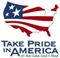Duration Plots

# Percentile definition

A percentile is a value on a scale of one hundred that indicates the percent of a distribution that is equal to or below it. For example, on the map of daily streamflow conditions a river discharge at the 90th percentile is equal to or greater than 90 percent of the discharge values recorded during all years that measurements have been made. In these plots, the percentiles are based on historic daily values calculated for each month. In general,
a percentile greater than 75 is considered above normal
a percentile between 25 and 75 is considered normal
a percentile less than 25 is considered below normal
In some hydrological studies, particularly those related to floods, a variation of the percentile known as the "percent exceedance" is used.  It is simply obtained by subtracting the percentile scale value  from 100 percent.  For example, a discharge at the 75th percentile is the same as a discharge at the 25th percent exceedance (100-75=25).U.S. Department of the Interior | U.S. Geological Survey
URL:
Page Contact Information: Virginia WSC Webmaster